Courses

# Capacitors & Inductors - 2

## 20 Questions MCQ Test Topicwise Question Bank for Electrical Engineering | Capacitors & Inductors - 2

Description
This mock test of Capacitors & Inductors - 2 for Electrical Engineering (EE) helps you for every Electrical Engineering (EE) entrance exam. This contains 20 Multiple Choice Questions for Electrical Engineering (EE) Capacitors & Inductors - 2 (mcq) to study with solutions a complete question bank. The solved questions answers in this Capacitors & Inductors - 2 quiz give you a good mix of easy questions and tough questions. Electrical Engineering (EE) students definitely take this Capacitors & Inductors - 2 exercise for a better result in the exam. You can find other Capacitors & Inductors - 2 extra questions, long questions & short questions for Electrical Engineering (EE) on EduRev as well by searching above.
QUESTION: 1

### There inductances, 1 H, 2 H and L H are in parallel. The maximum value of equivalent inductance will be

Solution: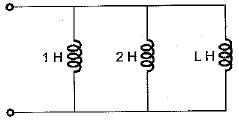Equivalent inductance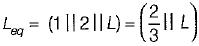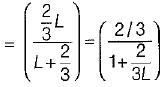Leq will be maximum of L → ∞
Hence,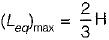QUESTION: 2

### Figure given below shows a parallel combination of 10 mH and 20 mH inductances in parallel. A time varying voltage is applied across the inductances at any time instant and the total current supplied by the time varying source is 25 amps. The values of instantaneous currents through each of the inductances are given by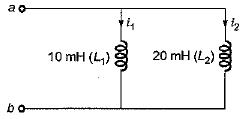Solution:

Let the applied voltage be v.
∴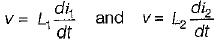or,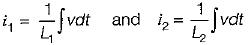∴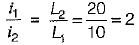or, i1 = 2 i2   ....(i)
Also, i1 + i2 = 25  ...(ii)
Solving equations (i) and (ii), we get
i= 16.67 A and i2 = 8.33A

QUESTION: 3

### The equivalent capacitance between the terminals a-b for the capacitive circuit shown below is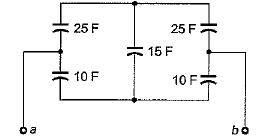Solution:

The given circuit can be redrawn as shown below.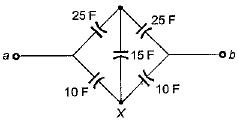The star at point X can be replaced by equivalent delta having delta brances as C1 , C2 and C3 as shown below.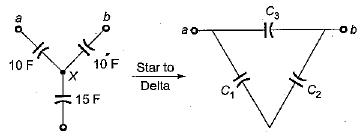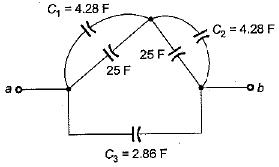Hence,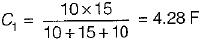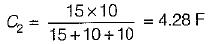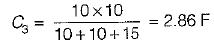Combining the parallel capacitances, the equivalent circuit reduces as shown below.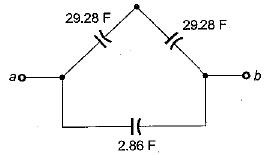Hence, equivalent capacitance between terminals a and b is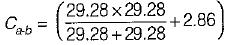= 17.5 F

QUESTION: 4

A voltage v(t) = e-t sin t is connected to a capacitance C = 2 F. The energy stored in the capacitor for t = 0 to t = 10 sec is

Solution:

Capacitive energy =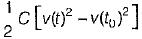Given, v(t) = sin t · e-t
∴ v(10) = (sin 10) · e-10 V
and v(0) = 0 V
∴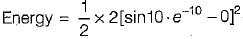= sin210 · e-20 Joules

QUESTION: 5

A sawtooth voltage as shown in figure below is applied between the terminals A-B to a capacitor of C = 30 μF. The current i will be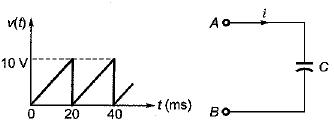Solution:

Given waveform is a periodic function having a period of 20 ms.
Here,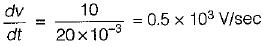∴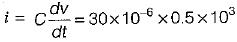= 15 mA

QUESTION: 6

Assertion (A): In steady state condition, the energy consumed is infinite for both inductor and capacitor.
Reason (R): The current i in the capacitor and voltage v\n the inductor are zero in steady state.

Solution:

Assertion is false because energy consumed is zero in steady state since capacitor acts as open circuit (i = 0) and inductor acts as short-circuit (v = 0) in steady state.

QUESTION: 7

Assertion (A): If the voltage across the capacitor being constant, current through it is zero.
Reason (R): On application of dc voltage and with no initial charge, the capacitor first acts as short circuit but as soon as the full charge it retains, the capacitor behaves as open circuit.

Solution:
QUESTION: 8

A 10 μF capacitor is charged full by 100 V dc source. It is then connected across an uncharged capacitor of 50 μF capacity. The energy stored in the two capacitors in the final state will be

Solution:

Given, C1 = 10μF
and C2 = 50 μF; V = 100 volt
Initial energy stored in the capacitor of 10 μF is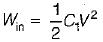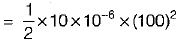= 0.05 Joules
The initial charge,
Qin = CV = 10 x 10-6 x 100
= 0.001 Coulomb
When this 10 μF capacitor is connected across the unchanged capacitor, let the final voltage be V' volt.
Then,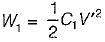and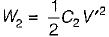Again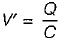where, Q is the final charge and C is the final capacitance.
Now, Q = Qin = 0.001 Columb
and Ceq = C - C1 + C2
= 10 + 50 = 60 μF
Hence, final voltage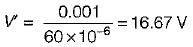Thus,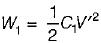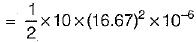= 0.0014 J = 1.4 mJ
and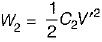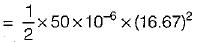= 0.007 J = 7 mJ

QUESTION: 9

For the bridge circuit shown below, the charge accumulated in the capacitor under steady state condition is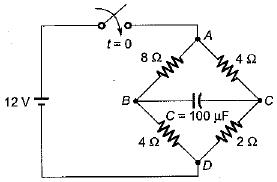Solution:

Under steady state condition, the capacitor will acts as open circuit.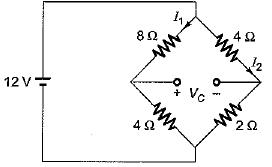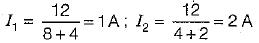Let the voltage across capacitor be VC in steady state.
Then, VC = - 8I1 + 4I2
= - 8 x 1 + 4 x 2
= - 8 + 8 = 0
Hence, charge accumulated in the capacitor is
Q = CV= (100 μF) x 0 = 0

QUESTION: 10

A current of i = 300t is suddenly applied to an inductance of 1 mH. The induced voltage will be

Solution:

Induced voltage,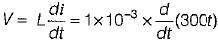= 10-3 x 300 - 0.3 volt

QUESTION: 11

A 100-turn coil has an inductance of 6 mH. if the number of turns is increased by 200%, all other quantities remaining the same, the inductance will be

Solution: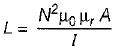∴ L ∝ N2
Since N is increased by 200% i.e. N is doubled therefore, new value of L is
L' = 4 X L
= 4 x 6 = 24 mH

QUESTION: 12

A current input,5 δ(t) is flow through a capacitor C. The voltage VC(t), across capacitor is given by

Solution: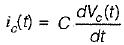or,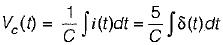Now,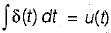So,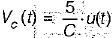QUESTION: 13

A voltage waveform v(t) = 12 t2 is applied across1 H inductor for t > 0 with initial current through it being zero. The current through the inductor for t > 0 is given by

Solution:

We know that,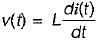or,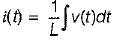or,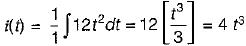QUESTION: 14

Which of the following statements associated with capacitor is wrong?

Solution:

Statement (b) holds true for an inductor not for a capacitor.

QUESTION: 15

Potential difference across a capacitor of capacitance of 20 μF is increased uniformly from 0 to 240 V in 1 second. The charging current will be

Solution: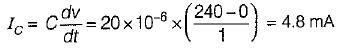QUESTION: 16

Assertion (A): Lower the self inductance of a coil more the delay in establishing steady current through it.
Reason (R): An inductor opposes a sudden change in current.

Solution:

Lower the self inductance of a coil less the delay in establishing steady current through it because lower will be the inductive reactance which will offer less opposition to the flow of current.
Hence, assertion is false.

QUESTION: 17

An open coil has

Solution: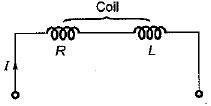A coil acts as open circuit i.e I = 0 if R = ∞  or L = 0 (so that XL = 0Ω).

QUESTION: 18

Consider the following statements regarding an inductor:
1. An inductor is a sort of short circuit to dc.
2. An inductor is a sort of open circuit to dc.
3. An inductor never dissipates energy but only stores it.
4. A finite amount of energy can be stored in an inductor even if voltage across it is zero, such as when the current through it is constant.
Q. Which of the above statements is/are not correct?

Solution:

An inductor acts as open circuit to dc (as f = 0 for dc so that XL = 0Ω).

QUESTION: 19

Assertion (A): A capacitor can store charge.
Reason (R): The plates of the condenser are conductive.

Solution:
QUESTION: 20

Assertion (A): It is impossible to change the current through an inductor by finite amount in zero time.
Reason (R): The current through an inductor can be changed by finite amount in zero time only when an infinite voltage is applied across it.

Solution: